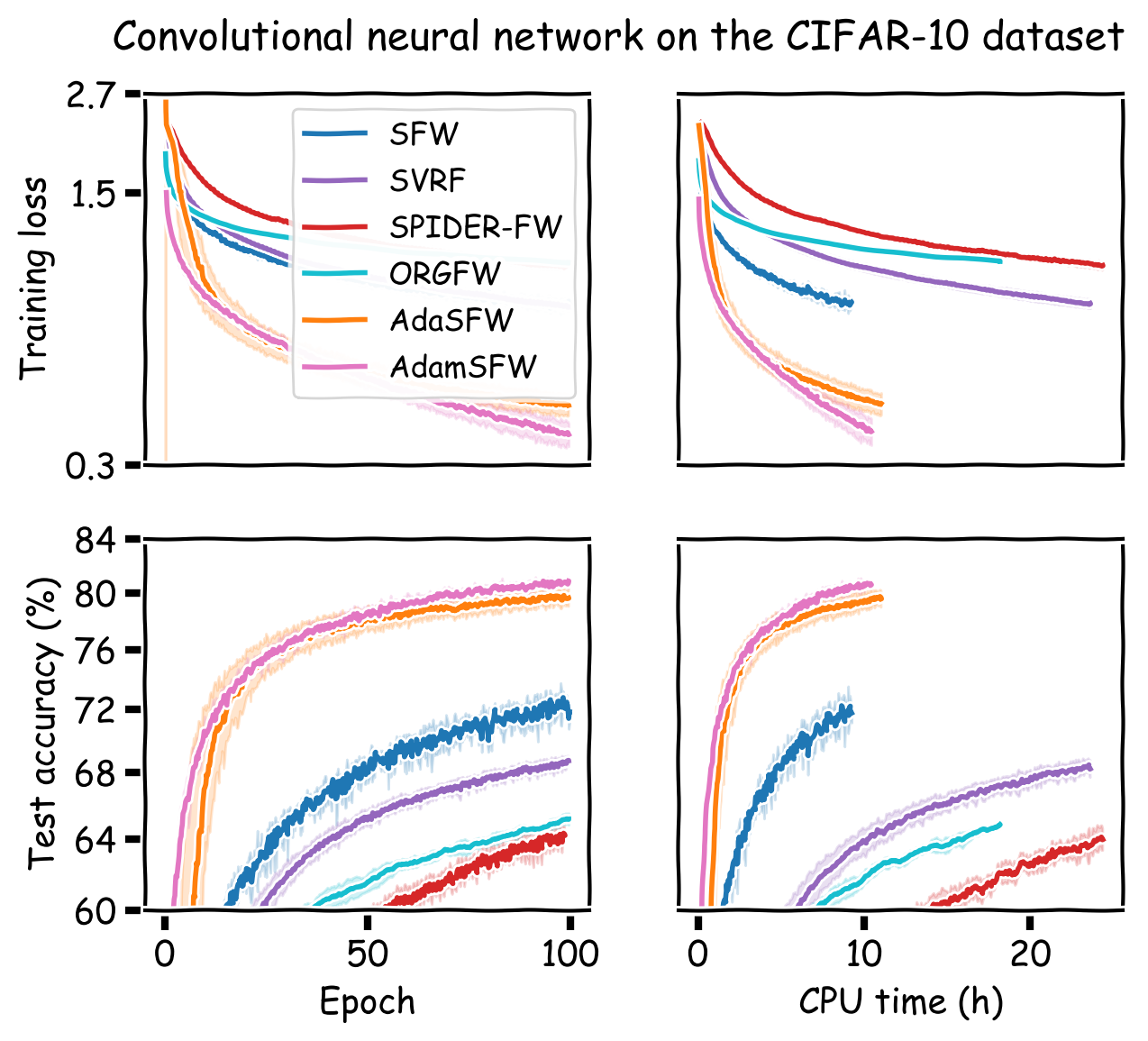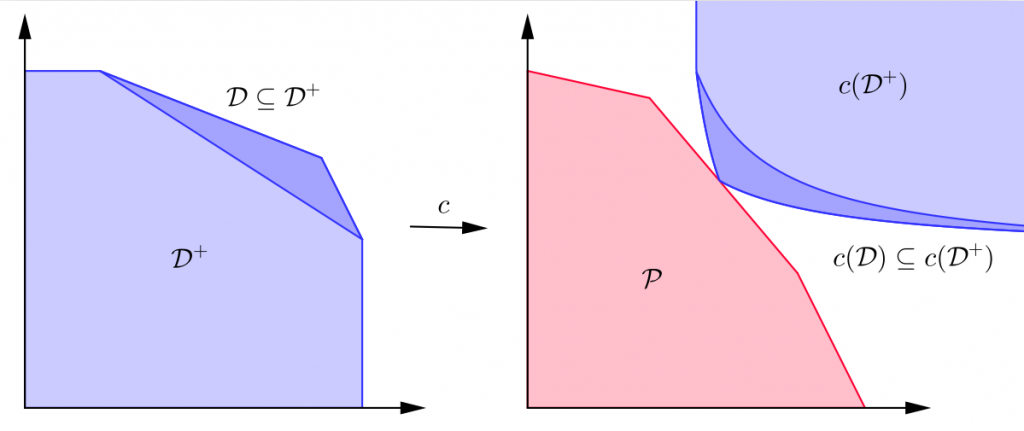# AA3 – Networks

## Beyond the Worst-Case: Data-Dependent Rates in Learning and Optimization

Sebastian Pokutta

Project Members

Project Duration

01.01.2021 − 31.12.2022

Located at

TU Berlin

### Description

Worst-case complexity bounds are increasingly insufficient to explain the (often superior) real-world performance of optimization and learning algorithms. We will consider data-dependent rates, approximation guarantees, and complexity bounds to provide guarantees much more in line with actual performance. We are in particular interested in exploiting properties of the feasible region to obtain algorithms that are adaptive to the problem structure.

One interesting instance of this situation is for the case of Carathéodory’s theorem. This theorem states that any point in the convex hull of of n points in the d-dimensional space is a convex combination of at most d+1 of those points. However, if approximate solutions are allowed, one can compute smaller combinations that are close enough to the target point . The size of this subset depends on the diameter of the convex hull.

This has applications for linear programs. Linear programs are one one of the most common classes of optimization problems that appear in scientific computing. It is currently unknown if these can be solved in strongly polynomial time, and the search for more efficient algorithms in practice is an active research field. The approximate Carathéodory’s theorem in the dual linear program setting states that the optimum dual solution of a linear program is a convex combination of at most d+1 of the constraints. Since real life linear programs are often not completely pathologic, techniques such as this exploiting the regularity of the data are an interesting research direction.

We also study the particular case for packing linear programs. Packing linear programs have all constraints and variables positive real numbers. We show that solving the 1-fair dual packing problem efficiently can be done efficiently exploiting the geometry of the input polytope. 

A more technical overview can be found [here].

External Website

Related Publications

1. Besançon, M., Carderera, A., and Pokutta, S. (2021). FrankWolfe.jl: a high-performance and flexible toolbox for Frank-Wolfe algorithms and Conditional Gradients. Preprint[arXiv] [code]
2. Combettes, C. W., and Pokutta, S. (2019). Revisiting the Approximate Carathéodory Problem via the Frank-Wolfe Algorithm. Preprint[arXiv] [summary] [slides] [code] [video]
3. Kerdreux, T., Roux, C., d’Aspremont, A., and Pokutta, S. (2021). Linear Bandits on Uniformly Convex Sets. Preprint[arXiv] [summary]
4. Carderera, A., Diakonikolas, J., Lin, C. Y., and Pokutta, S. (2021). Parameter-free Locally Accelerated Conditional Gradients. Preprint[arXiv]
5. Combettes, C. W., Spiegel, C., and Pokutta, S. (2020). Projection-Free Adaptive Gradients for Large-Scale Optimization. Preprint[arXiv] [summary] [code]
6. Kerdreux, T., d’Aspremont, A., and Pokutta, S. (2021). Local and Global Uniform Convexity Conditions. Preprint[arXiv]
7. Criado, F, Martínez-Rubio, D, and Pokutta, S. (2021). Fast Algorithms for Packing Proportional Fairness and its Dual. Preprint [arXiv]

Related Pictures